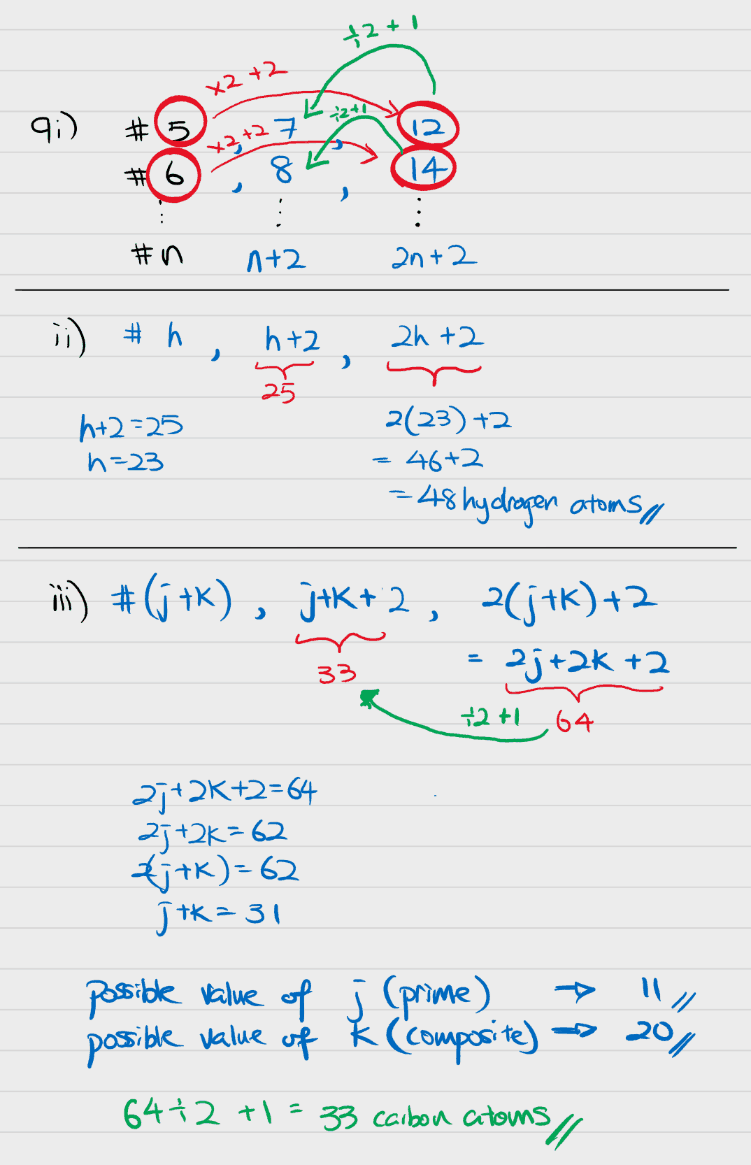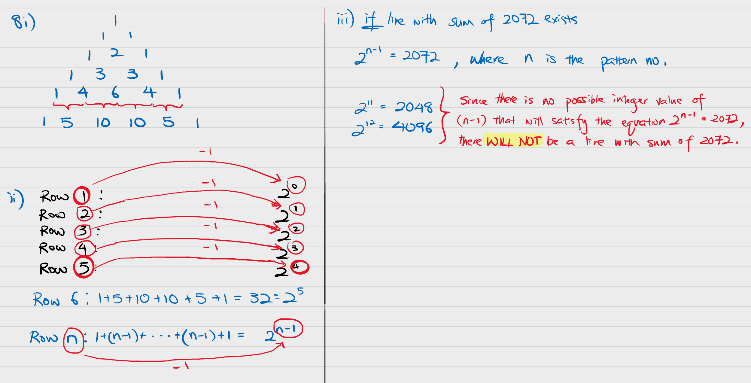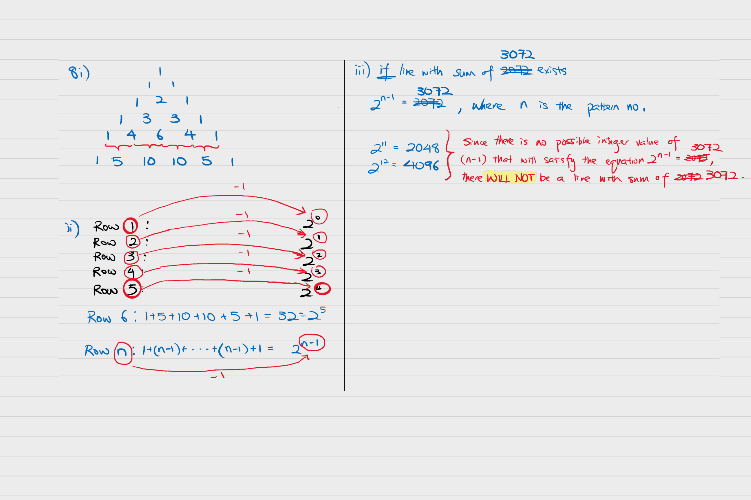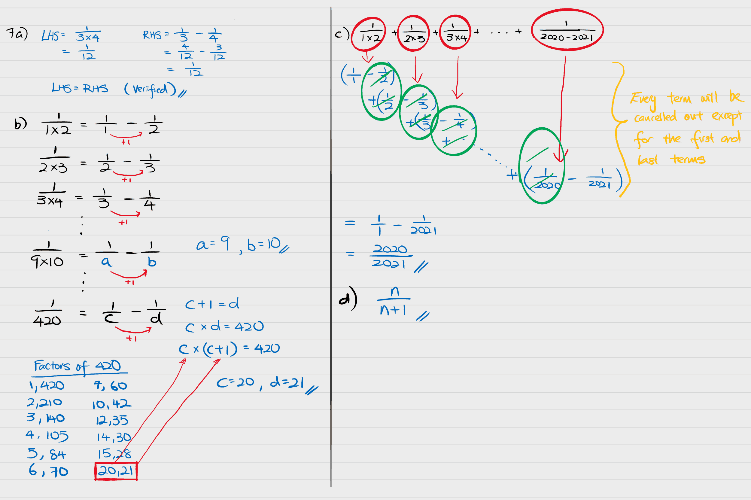# Answer0 Replies 1 Like ✔Accepted Answer1 Reply 1 LikeOoops, how careless of me. Have amended as per picture.

However, the answer remains the same in that there will not be a line with the sum of 3072.

For a sum to appear on the pattern, it must be able to be expressed as 2 to the power of something, where that something must be an integer. Since no such integer value exists (2 to the power of 11 is 2048 and 2 to the power of 12 is 4096) that will result in a sum of 3072, that means there will not be any line with a sum of 3072.

1 Reply 1 Like1 Reply 1 Like